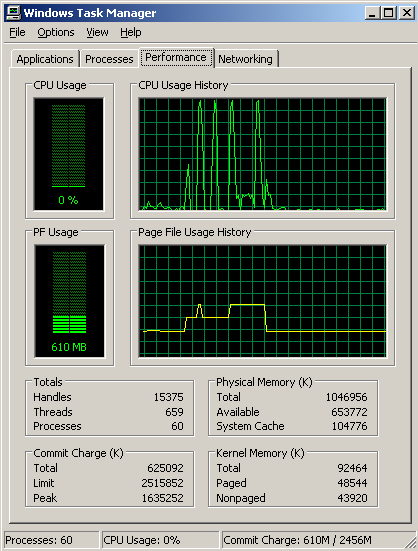# In-place Operations on Data

Recently I mentioned that I would describe a relatively new optimization we have for MATLAB code that allows some function calls to operate on variables without allocating more memory for the result. This can be very beneficial when processing large datasets. I will describe and demonstrate this feature using R2007a.

### Make Data

Create a "large" dataset. This one works for me with 1GB RAM and 1.5GB of swap space running Windows XP and no other applications.

n = 38*2^20;
x = randn(n,1);

Now call another function that will call either a regular function or an in-place function.

inplaceTest(x)

Let's look at the code for inplaceTest.

type inplaceTest
function inplaceTest(x)
% Call functions with either regular or in-place semantics.

%% Call a Regular Function with the Same Left-Hand Side
x = myfunc(x);
%% Call an In-place Function with the Same Left-Hand Side
x = myfuncIP(x);
%% Call a Regular Function with a Different Left-Hand Side
y = myfunc(x);
%% Call an In-place Function with Same Left-Hand Side
% Note: if we changed this next call to assign output to a new LHS, we get an error
x = myfuncIP(x);


It just gets the data in and twice calls two different functions.

See what the Windows Task Manager shows for a recent run of mine for the 4 calculations. You can see when the CPU was busy and you can match that with the times that memory was allocated, whether temporary or permanent. Here's how a typical run of this code appears in the Windows Task Manager Performance tab when I run it.You can see the four calls to the computational functions in the CPU Usage History, and you can see the amount of extra memory used in the Page File Usage History. When we call the in-place function using the same input and output variable names from the test function, no extra memory is allocated.

### Required Characteristics of In-place Behavior

Let's look at the code for the two functions myfunc and myfuncIP so we can see how they differ.

Here's the function myfunc.m

type myfunc
function y = myfunc(x)
y = sin(2*x.^2+3*x+4);


and here's the function myfuncIP.m

type myfuncIP
function x = myfuncIP(x)
x = sin(2*x.^2+3*x+4);


As you can see, the two myfunc functions differ. The in-place version has a return argument with the same name as one of the input arguments, in this case, x. Also, the computation needs to put its results into x instead of y, since the new return variable name is now different.

The second important idea required to take advantage of in-place computation is that the in-place function must itself be called from another function, as I've done with inplaceTest.

### Considerations

I'd like to pass on several tips and rules of thumb about in-place functions. This is not an ordered list.

• Because of MATLAB's JIT, some functions or parts of code are already doing some work in place. In these cases, you will see less or no benefit than you might expect by switching to in-place operations. For example, using the binary version of max (e.g, A=max(A,B)) doesn't gain, even if in a function.
• Benefits don't usually occur for the smallest arrays. It takes typically at least 1000 elements before you might see a difference.
• Keep your code natural. Don't write unnatural code just to take advantage of this. This is particularly true if your function is already computationally intensive. There is more speed gain for simpler functions, though memory might still be a concern. For example, lu is an order n^3 operation, and the memory speed gain is only order n^2. So it's not worth doing contortions to write [L A P] = lu(A) (more naturally written [L U P] = lu(A)), unless you really need to conserve memory.

### Limitations

There are some limitations regarding when the in-place optimization is operative. These include:

• Not all built-in MATLAB functions currently obey in-place semantics. We tried to support the most important and obvious ones first such as elementwise operations. There is not a list of these functions. It will grow from release to release.
• There is no interface for the in-place operations via MEX-files.
• MATLAB should be able to recognize in-place possibilities even when variable names don't match between the input and output names.

### Feedback?

Do you plan to take advantage of this new feature in your code? Let me know here.

Published with MATLAB® 7.4

|